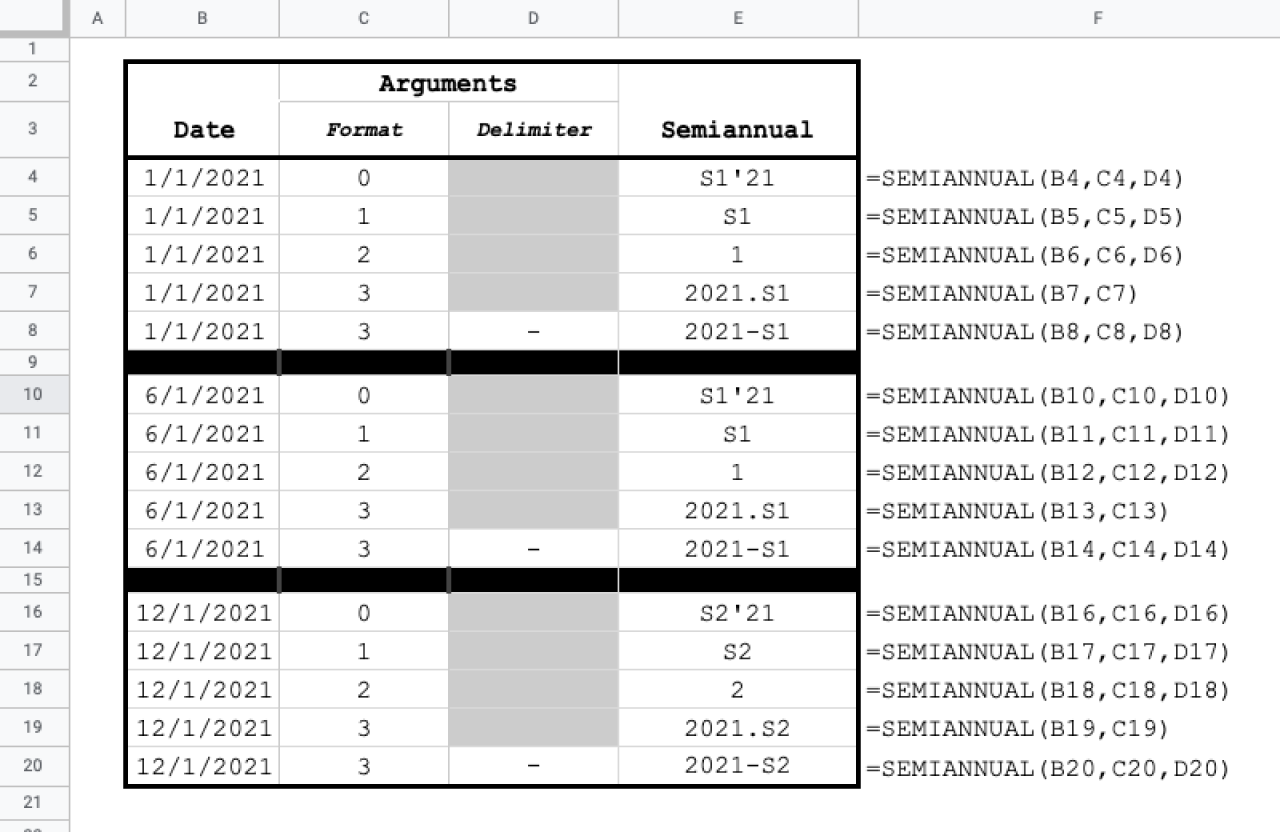# Transform dates to financial half year in Google Sheets with SEMIANNUAL

Jul 7

The SEMIANNUAL function is an essential tool for pivoting and grouping data by half year.

### Function Signature

`=SEMIANNUAL(input, format, delimiter)`

### Argument Definitions

[range of dates] input; required - date or range of dates to convert. If string or number is passed, the function attempts to convert to a date.

[number] format - how to generate combinations

• 0 - S1'YY or S2'YY [default]
• 1 - S1 or S2
• 2 - 1 or 2 for first-half and second-half of the year
• 3 - yyyy.S1 or yyyy.S2

[string] delimiter - also known as the separator for items in a given combination

## Use the Cheat

Click the Code Box to Copy

Cheat copied to clipboard ✂️👍
``` /** * Returns half-year for a date. * * @param {array} input - date or range of dates * @param {int} format - 0 by default * 0 returns S1'YY or S2'YY * 1 returns S1 or S2 * 2 returns a number 1 or 2 * 3 returns yyyy.S1 or yyyy.S2 * * @param {string} delimiter - separator for return format 3 only * * @customfunction * */ function SEMIANNUAL(input, format = 0, delimiter = "."){ const is_array = input instanceof Array; let ret = [[]]; if (input instanceof Array) { const is_matrix = input.length > 1; for (let i=0; i < input.length; i++) { if (is_matrix) { for (let j=0; j < input[i].length; j++) { if (!ret[i]) { ret[i] = []; } ret[i].push(single_semiannual_(input[i][j], format, delimiter)); } } else { if (!ret[i]) { ret[i] = []; } ret[i].push(single_semiannual_(input[i], format, delimiter)); } } } else { ret = [single_semiannual_(input, format, delimiter)]; } return ret; }; function single_semiannual_(input, format = 0, delimiter = '.') { if (!(input instanceof Date)) { input = new Date(input); } const s = input.getSemiannual(); const year = input.getFullYear(); switch(format) { case 1: return "S" + s; case 2: return s; case 3: return year.toString() + delimiter + "S" + s; }; // default case let year_str = year.toString(); if (year < 1000) { return "The year is prior to 1000 AD. This looks like an error. If not, email us at support@lassie.ai to tell us what's up."; } return "S" + s + "'" + year_str.substring(year_str.length-2, year_str.length); } Date.prototype.getSemiannual = function() { let month = this.getMonth(); return Math.floor(month / 6) + 1; }; ```Cyrus Radfar